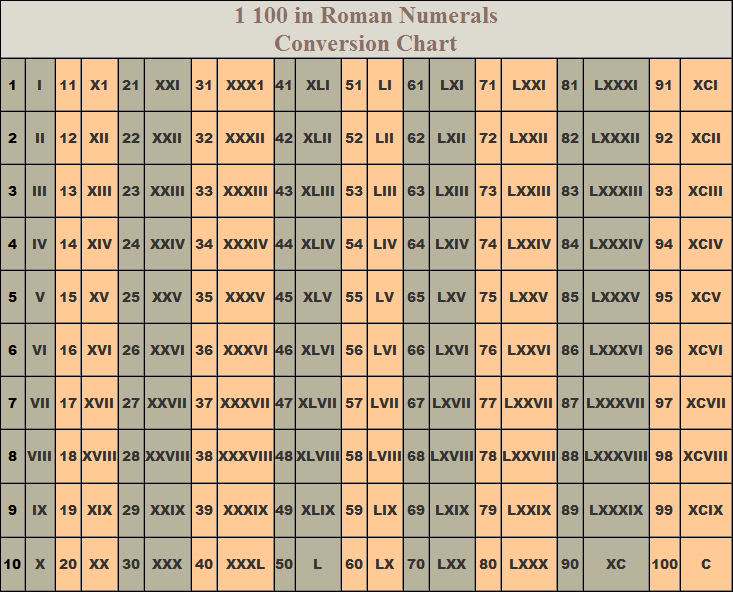Home Education The Roman Numerals

# The Roman Numerals

62The numeric system represented by Roman numerals originated in ancient Rome and remained the usual way of writing numbers throughout Europe well into the Late Middle Ages. Numbers in this system are represented by combinations of letters from the Latin alphabet. Roman numerals, as used today, are based on seven symbols:

1. I – 1
2. V – 5
3. X – 10
4. L – 50
5. C – 100
6. D – 500
7. M – 1000

Basic decimal pattern

The original pattern for Roman numerals used the symbols I, V. and X (1, 5, and 10) as simple tally marks. Each marker for 1 (I) added a unit value up to 5 (V), and was then added to (V) to make the numbers from 6 to 9:

I, II, III, IIII, V, VI, VII, VIII, VIIII, X.

The numerals for 4 (IIII) and 9 (VIIII) proved problematic (among other things, they are easily confused with III and VIII), and are generally replaced with IV (one less than 5) and IX (one less than 10). This feature of Roman numerals is called subtractive notation.

The numbers from 1 to 10 (including subtractive notation for 4 and 9) are expressed in Roman numerals as follows:

I, II, III, IV, V, VI, VII, VIII, IX, X.

The system being basically decimal, tens and hundreds follow the same pattern:

Thus 10 to 100 (counting in tens, with X taking the place of I, L taking the place of V and C taking the place of X):

X, XX, XXX, XL, L, LX, LXX, LXXX, XC, C.

Note that 40 (XL) and 90 (XC) follow the same subtractive pattern as 4 and 9.

Similarly, 100 to 1000 (counting in hundreds):

C, CC, CCC, CD, D, DC, DCC, DCCC, CM, M.

Note: The use of Roman numerals continued long after the decline of the Roman Empire. From the 14th century on, Roman numerals began to be replaced in most contexts by the more convenient Hindu-Arabic numerals; however, this process was gradual, and the use of Roman numerals persists in some minor applications to this day.

Previous articleDistance From Ogaminana To Umuahia
Next articleDistance From Ogaminana To Uromi